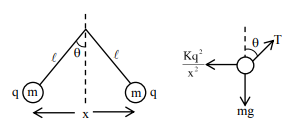# Two identical tennis balls each having mass 'm' and charge 'q' are suspended from a fixed point by threads of length 'l'.Question:

Two identical tennis balls each having mass 'm' and charge 'q' are suspended from a fixed point by threads of length 'l'. What is the equilibrium separation when each thread makes a small angle '' with the vertical ?

1. $\mathrm{x}=\left(\frac{\mathrm{q}^{2} l}{2 \pi \varepsilon_{0} \mathrm{mg}}\right)^{\frac{1}{2}}$

2. $x=\left(\frac{q^{2} l}{2 \pi \varepsilon_{0} m g}\right)^{\frac{1}{3}}$

3. $x=\left(\frac{q^{2} l^{2}}{2 \pi \varepsilon_{0} m^{2} g}\right)^{\frac{1}{3}}$

4. $x=\left(\frac{q^{2} l^{2}}{2 \pi \varepsilon_{0} m^{2} g^{2}}\right)^{\frac{1}{3}}$

Correct Option: , 2

Solution:$\mathrm{T} \cos \theta=\mathrm{mg}$

$\mathrm{T} \sin \theta=\frac{\mathrm{kq}^{2}}{\mathrm{x}^{2}}$

$\tan \theta=\frac{k q^{2}}{x^{2} m g}$

as $\tan \theta \approx \sin \theta \approx \frac{x}{2 L}$

$\frac{\mathrm{x}}{2 \mathrm{~L}}=\frac{\mathrm{Kq}^{2}}{\mathrm{x}^{2} \mathrm{mg}}$

$x=\left(\frac{q^{2} L}{2 \pi \varepsilon_{0} m g}\right)^{1 / 3}$